# ICSE Solutions for Selina Concise Chapter 9 Triangles Class 9 Maths

### Exercise 9(A)

1. Which of the following pairs of triangles are congruent? In each case, state the condition of congruency:

(a) In ΔABC and ΔDEF, AB = DE, BC = EF and B = E.

(b) In ΔABC and ΔDEF, B = E = 90o; AC = DF and BC = EF.

(c) In ΔABC and Δ QRP, AB = QR, B = R and C = P.

(d) In ΔABC and Δ PQR, AB = PQ, AC = PR and BC = QR.

(e) In ΔADC and Δ PQRΔ, BC = QR, A = 90o, C = R = 40o and Q = 50o.

( f) In Triangle ABC and Triangle BCD, ∠ABC = ∠DCB (each = 90°) and ∠A =40°

(a)

In ⧍ABC and ⧍DEF

AB = DE [Given]

∠B = ∠E [Given]

BC = EF [Given]

By Side - Angle - Side criterion of congruency, the triangles ⧍ABC and ⧍DEF are congruent to each other .

∴ ∆ABC ≅ DEF

(b)

In ∆ABC and ∆DEF
∠B = ∠E = 90°
Hyp. AC = Hyp.DF
BC = EF

By Right Angle – Hypotenuse – Side criterion of congruency, the triangles ∆ABC and ∆DEF are congruent to each other.
∴ ∆ABC ≅ ∆DEF

(c)
In ∆ABC and ∆QRP
∠B =∠R [Given]
∠C = ∠P [Given]
AB = QR [Given]

By Angle – Angle – Side criterion of congruency, the triangles ∆ABC and ∆QRP are congruent to each other.
∴ ∆ABC ≅ ∆QRP

(d)
In ∆ABC and ∆PQR
AB = PQ [Given]
AC = PR [Given]
BC = QR [Given]

By Side – Side – Side criterion of congruency, the triangles ∆ ABC and ∆PQR are congruent to each other.
∴ ∆ABC ≅ ∆PQR

(e)
In ∆PQR
∠R = 40°, ∠Q = 50°
∠P +∠Q+∠R = 180° [Sum of all the angles in a triangle = 180°]
⇒ ∠P +50° + 40° = 180°
⇒ ∠P + 90° = 180°
⇒ ∠P = 180°−90°
⇒ ∠P = 90°
In ∆ ABC and ∆ PQR
∠ A = ∠P
∠C = ∠R
BC =QR

By Angel –Angel –Side criterion of congruency, the triangles ∆ABC and ∆PQR are congruent to each other.
∴ ∆ABC ≅ ∆PQR

(f)
In ∆ABC and ∆DCB
∠ABC = ∠DCB (each = 90°)
Hyp. AC = Hyp. BD
side BC = Side BC (common)

∴ ∆ABC ≅ ∆DCB (R.H.S. axiom of congruency)

2. The given figure shows a circle with centre O. P is mid-point of chord AB.

Show that OP is perpendicular to AB

Given : In the figure, O is centre of the circle, and AB is chord. P is a point of AB such that AP = PB.
We need to prove that, OP⟂AB

Construction : Join OA and OB
Proof:
In △OAP and △OBP
OA = OB [radii of the same circle]
OP = OP [common]
AP = PB [given]
∴ By Side - Side - Side criterion of congruency,
△OAP ≅ △OBP
The corresponding parts of the congruent triangles are congruent.
∴ ∠OPA = ∠OPB  [by c.p.c.t]
But ∠OPA + ∠OPB = 180° [linear pair]
∴ ∠OPA = ∠OPB = 90°
Hence OP ⟂ AB

3. The following figure shows a circle with centre O.

If OP is perpendicular to AB, prove that AP = BP.

Given: In the figure, O is centre of the circle, and AB  is chord. P is a point of AB uch that AP = PB.
we need to prove that , AP = BP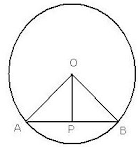Construction : Join OA and OB
Proof :
In right triangles △OAP and △OBP
Hypotenuse OA = OB [radii of the same circle]
Side OP =OP [common]

∴ By Right Angle -Hypotenuse -Side criterion of congruency,
△OAP ≅ △OBP
The corresponding parts of the congruent triangles are congruent.
∴ AP = BP [by c.p.c.t]
Hence, proved.

4. In a triangle ABC, D is mid-point of BC; AD is produced upto E so that DE = AD. Prove that:

(i) ABD and ECD are congruent.

(ii) AB = CE.

(iii) AB is parallel to EC.

Given : A △ABC in which D is the mid - point of BC.
We need to prove that
(i) △ABD ≅ △ECD
(ii) AB = EC
(iii) AB ∥ EC

(i) In △ABD nd △ECD
BD = DC  [D is the midpoint of BC]
∠ADB = ∠CDE  [vertically opposite angles]
∴ By Side - Angle - Side criterion of congruence, we have,
△ABD ≅ △ECD
(ii) The corresponding parts of  the congruent triangles are congruent.
∴ AB = EC [c.p.c.t]
(iii) Also, ∠DAB = ∠DEC [c.p.c.t]
AB ∥ EC [∠DAB and ∠DEC are alternate angles]

5. A triangle ABC has B = C.

Prove that:

(i) The perpendiculars from the mid-point of BC to AB and AC are equal.

(ii) The perpendiculars form B and C to the opposite sides are equal.

(i) Given: A △ABC in which ∠B = ∠C.
DL is the perpendicular from D to AB
DM is the perpendicular from D to AC

We need to prove that
DL = DM
Proof:
In △DLB and △DMC
∠DLB = ∠DMC = 90° [DL ⟂AB and DM ⟂ AC]
∠B = ∠C [Given]
BD = DC [D is the midpoint of BC]
∴ By Angle - Angle - Side criterion of congruence,
△DLB ≅ △DMC
The corresponding parts of the congruent triangles are congruent.
∴ DL = DM  [c.p.c.t]
(ii) Given : A △ABC in which ∠B = ∠C .
BP is the perpendicular from D to AC
CQ is the perpendicular from C to ABWe need to prove that
BP = CQ
Proof :
In △BPC and △CQB
∠B = ∠C [Given]
∠BPC = ∠CQB = 90°  [BP ⟂ AC and CQ⟂AB]
BC = BC [Common]
∴ By Angle - Angle - Side criterion of congruence,
△BPC ≅ △CQB
The corresponding parts of the congruent triangles are congruent.
∴ BP = CQ [c.p.c.t]

6. The perpendicular bisectors of the sides of a triangle ABC meet at I.

Prove that: IA = IB = IC.

Given : A △ABC in which AD is the perpendicular bisector of BC
BE is the perpendicular bisector of CA
CF is the perpendicular bisector of AB
AD, BE and CF meet at I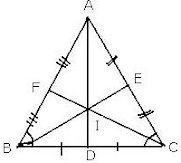We need to prove that
IA = IB = IC
Proof :
In △BID and △CID
BD = DC [Given]
∠BDI  = ∠CDI = 90° [AD is the perpendicular bisector of BC]
BC = BC [Common]
∴ By Side - Angle - Side criterion of congruence,
△BID ≅ △CID
The corresponding parts of the congruent triangles are congruent.
∴ IB = IC [c.p.c.t]
Similarly,
In △CIE and △AIE
CE = AE  [Given]
∠CEI = ∠AEI = 90° [AD is the perpendicular bisector of BC]
IE = IE [Common]
∴ By Side - Angle - Side criterion of congruence,
△CEI ≅ △AIE
The corresponding parts of the congruent triangles are congruent.
∴ IC = IA  [c.p.c.t]
Thus, IA = IB = IC

7. A line segment AB is bisected at point P and through point P another line segment PQ, which is perpendicular to AB, is drawn. Show that: QA = QB. Answer

Given : A △ABC in which AB is bisected at P
PQ is perpendicular to AB

We need to prove that
QA = QB
Proof :
In △APQ and △BPQ
AP = PB  [P is the mid - point of AB]
∠APQ = ∠BPQ = 90° [PQ is perpendicular to AB]
PQ = PQ [Common]
∴ By Side - Angle - Side criterion of congruence,
△APQ ≅ △BPQ
The corresponding parts of the congruent triangles are congruent.
∴ QA = QB  [c.p.c.t]

8. If AP bisects angle BAC and M is any point on AP, prove that the perpendiculars drawn from M to AB and AC are equal. Answer

From M, draw ML such that ML is perpendicular to AB and MN is perpendicular to ACIn △ALM and △ANM
∠LAM=∠MAN  [AP is the bisector of ∠BAC]
∠ALM=∠ANM=90° [ML  AB, MN AC]
AM = AM [Common]
∴ By Angle-Angle-Side criterion of congruence,
△ALM = △ANM
The corresponding parts of the congruent triangles are congruent.
ML=MN [c.p.c.t]
Hence proved.

9. From the given diagram, in which ABCD is a parallelogram, ABL is al line segment and E is mid point of BC.

Prove that:

(i) DCE LBE

(ii) AB = BL.

(iii) AL = 2DC

Given: ABCD is a parallelogram in which E is the mid-point of BC.
We need to prove that
(ii) AB = BL
(iii) AL = 2DC

(i) In △DCE and △LBE
∠DCE = ∠EBL  [DC ||AB, alternate angles]
CE=EB  [E is the midpoint of BC]
∠DEC= ∠LEB  [vertically opposite angles]
By Angle-Side-Angle criterion of congruence, we have,
△DCE = △LBE
The corresponding parts of the congruent triangles are congruent.
DC = LB  [c.p.c.t] ...(1)

(ii) DC=AB [opposite sides of a parallelogram]...(2)
From (1) and (2),
AB=BL ...(3)

(iii) AL = AB+BL ...(4)
From (3) and (4),
AL=AB+AB
AL = 2AB
AL = 2DC [from (2)]

10. In the given figure, AB = DB and AC = DC.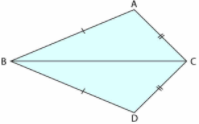If ABD = 58o,

DBC = (2x – 4)o,

ACB = y + 15o and

DCB = 63o ; find the values of x and y.

Given: In the figure, AB=DB, AC=DC, ∠ABD=58°,

∠DBC=(2x- 4)°, ∠ACB = (y +15)° and ∠DCB= (63°)
We need to find the values of x and y.

In △ABC and △DBC
AB = DB [given]
AC=DC [given]
BC = BC [common]
∴ By Side-Side-Side criterion of congruence, we have,
△ABC = △DBC
The corresponding parts of the congruent triangles are congruent.
∠ACB=∠DCB [c.p.c.t]
⇒ y° + 15°=63°
⇒ y°=63° - 15°
⇒ y°=48°
and ∠ABC =∠DBC [c.p.c.t]
But, ∠DBC = (2x - 4)°
We have ∠ABC+∠DBC=∠ABD
⇒ (2x - 4)° + (2x - 4)° = 58°
⇒ 4x - 8° = 58°
⇒ 4x = 58° + 8°
⇒ 4x = 66°
⇒ x = 66°/4
⇒ x = 16.5°
Thus the values of x and y are :
x = 16.5° and y = 48°

11. In the given figure: AB||FD, AC||E and BD = CE; prove that:

(i) BG = DF

(ii) CF = EG

In the given figure AB ∥ FD,
⇒ ∠ABC =∠FDC
Also, AC ∥ GE,
⇒ ∠ACB=∠GEB

Consider the two triangles △GBE and △FDC
∠B=∠D
∠C=∠E
Also given that
BD = CE
⇒ BD+DE=CE+DE
⇒ BE = DC
By Angle - Side - Angle criterian of congruence
△GBE ≅ △FDC
GB/FD = BE/DC = GE/FC
But BE =DC
⇒ BE/DC = BE/BE  =  1
GB/FD =  BE/DC = 1
⇒ GB = FD
GE/FC =  BE/DC
⇒ GE = FC

12. In ∆ABC, AB = AC. Show that the altitude AD is median also.
AB = AC (Since △ABC is an isosceles triangle)
BD = DC (c.p.c.t)

In △DLB and △DMC,
BL = CM
(given)
∠DLB = ∠DMC (Both are 90°)
∠BDL = ∠CDM (vertically opposite angles)
∴△DLB ≅△DMC (AAS congruence criterion)
BD = CD (c.p.c.t)
Hence, AD is the median of LABC.

14. In the following figure, AB = AC and AD is perpendicular to BC. BE bisects angle B and EF is perpendicular to AB.

Prove that :

(i) BD = CD

(ii) ED = EF

AB = AC (given)
⇒ BD = CD (cpct)

(ii) In △EFB and △EDB,
∠EFB = ∠EDB (both are 90°)
EB = EB (common side)
∠FBE = ∠DBE (given)
∴ △EFB ≅ △EDB (PAS congruence criterion)
⇒ EF = ED (cpct)
that is, ED = EF.

15. Use the information in the given figure to prove :

(i) AB = FE

(ii) BD = CF

In △ABC and AEFD,
AB ∥ EF ⇒ ∠ABC = ∠EFD (alternate angles)
AC = ED (given)
∠ACB = ∠EDF (given)
∴ △ABC ≅ △EFD (AAS congruence criterion)
⇒ AB = FE (c.p.c.t)
and BC = DF (c.p.c.t)
BD + DC = CF+ DC (B-D -C-F)
BD = CF

### Exercise 9(B)

1. On the sides AB and AC of triangle ABC, equilateral triangle ABD and ACE are drawn.

Prove that: (i) CAD = BAE (ii) CD = BE.

Given : △ABD is an equilateral triangle
△ACE is an equilateral triangle
We need to prove that
(ii) CD = BEProof :
(i)

△ABD is equilateral
∴ Each angle = 60°
Similarly,
△ACE is equilateral
∴ Each angle = 60°
⇒ ∠CAE = 60° ....(2)
⇒ ∠BAD = ∠CAE  [from (1) and (2)] ....(3)
Adding ∠BAC to both sides, we have
∠BAD + ∠BAC = ∠CAE + ∠BAC

AC = AE [△ACE is equilateral]
AD = AB  [△ABD is equilateral]
∴ By Side - Angle- Side criterion of congruency,
The corresponding parts of the congruent triangles are congruent.
∴ CD = BE [by c.p.c.t]
Hence, proved.

2. In the following diagrams, ABCD is a square and APB is an equilateral triangle.

In each case,

(i) Prove that: .ΔAPD = Δ BPC

(ii) Find the angles of .Δ DPC

Given: ABCD is a square and △APB is an equilateral triangle.
We need to
(i) Prove that, △APD ≅ △BPC
(ii) To find angles of △DPC

(a)
(i) Proof :

AP = PB = AB  [△APB is an equilateral triangle]
Also, we have,
∠PBA = ∠PAB =∠APB = 60° ...(i)
Since ABCD is a square, we have
∠A = ∠B =∠C =∠D = 90° ....(2)
Since,
∠DAP  = ∠A - ∠PAB ...(3)
⇒ ∠DAP = 90°- 60°
⇒ ∠DAP  = 30°  [from (1) and (2)] ...(4)
Similarly,
∠CBP = ∠B - ∠PBA
⇒ ∠CBP = 90° - 60°
⇒ ∠CBP = 30°  [from (1) and (2)] ...(5)
⇒ ∠DAP = ∠CBP [from (4) and (5)] ...(6)
In △APD and △BPC
AD = BC  [Sides of square ABCD]
∠DAP = ∠CBP  [from (6)]
AP = BP  [Sides of equilateral △APB]
∴ By Side - Angle - Side criterion of congruence, we have,
△APD ≅ △BPC

(ii) AP = PB = AB  [△APB is an equilateral triangle] ...(7)
AB = BC = CD = DA  [Sides of square ABCD]....(8)
From (7) and (8), we have
AP = DA and PB = BC  ...(9)
In △APD,
AP = DA  [from (9)]
∴ ∠ADP = ∠APD  [Angles opposite to equal sides are equal]...(10)
∠ADP + ∠APD +∠DAP = 180°  [Sum of angles of a triangle = 180°]
⇒∠ADP + ∠ADP + 30° = 180°  [from (3), ∠DAP = 30° from (10), ∠ADP = ∠APD]
⇒ ∠ADP + ∠APD = 180° - 30°
We have,
⇒ ∠PDC = 90° - 75°
⇒ ∠PDC = 15° ...(11)
In △BPC,
PB = BC  [from (9)]
∴ ∠PCB = ∠BPC  [Angles opposite to equal sides are equal] ...(12)
∠PCB + ∠BPC + ∠CBP = 180°  [Sum of angles of a triangle = 180°]
⇒ ∠PCB + ∠PCB + 30° = 180° [from (5), ∠CBP = 30° from (12), ∠PCB = ∠BPC]
⇒ 2∠PCB = 180° - 30°
⇒ ∠PCB = 150°/2
⇒ ∠PCB = 75°
We have,
∠PCD = ∠C - ∠PCB
⇒  ∠PCD = 90° - 75°
⇒ ∠PCD = 15°
In △BPC,
PB = BC [from (9)]
∴ ∠PCB = ∠BPC  [Angles opposite to equal sides are equal] ...(12)
∠PCB + ∠BPC + ∠CBP = 180° [Sum of angles of a triangle = 180°]
⇒   ∠PCB+∠PCB + 30° = 180°  [from (5), ∠CBP = 30° and from (12) ∠PCB = ∠BPC]
⇒ 2∠PCB = 180° - 30°
⇒ ∠PCB = 150°/2
⇒ ∠PCB = 75°
We have,
∠PCD = ∠C - ∠PCB
⇒ ∠PCD = 90° - 75° = 15° ...(13)
In △DPC,
∠PDC = 15°
∠PCD = 15°
∠PCD + ∠PDC + ∠DPC = 180°  [Sum of angles of a triangle = 180°]
⇒ 15° +15°  + ∠DPC  = 180°
⇒ ∠DPC = 180° - 30°
⇒ ∠DPC =150°
∴ Angles of △DPC, are : 15°, 150°, 15°

(b)
(i) Proof: In △APB
AP = PB = AB  [△APB is an equilateral triangle]
Also, we have,
∠PBA = ∠PAB = ∠APB = 60° ...(1)
Since ABCD is a square, we have
∠A = ∠B =∠C = ∠D = 90°  ....(2)
Since ∠DAP = ∠A + ∠PAB ...(3)
⇒ ∠DAP = 90° + 60°
⇒ ∠DAP = 150°  [from (1) and (2)] ...(4)
Similarly ∠CBP = ∠B + ∠PBA
⇒ ∠CBP = 90° + 60°)

⇒ ∠CBP = 150°  [from (1) and (2)]...(5)
⇒ ∠DAP = ∠CBP   [from (4) and (5)] ....(6)
In △APD and △BPC
AD = BC  [Sides of square ABCD]
∠DAP = ∠CBP  [from (6)]
AP = BP  [Sides of equilateral △APB]
∴ By Side - Angle - Side criterion of congruence, we have,
△APD ≅ △BPC

(ii) AP = PB = AB  [△APB is an equilateral triangle ]...(7)
AB = BC = CD = DA  [Sides of square ABCD]...(8)
From (7) and (8), we have
AP = DA and PB = BC ...(9)
In △APD,
AP = DA  [from (9)]
∴ ∠ADP = ∠APD  [Angles opposite to equal sides are equal] ...(10)
∠ADP + ∠APD + ∠DAP = 180°  [Sum of angles of a triangle = 180°]
We have,
⇒∠PDC = 90° - 15°
⇒∠PDC = 75° ....(11)
In △BPC,
PB = BC  [from (9)]
∴ ∠PCB = ∠BPC  [ Angles opposite to equal sides are equal] ...(12)
∠PCB +∠BPC + ∠CBP = 180°   [Sum of angles of a triangle = 180°]
⇒ ∠PCB +∠PCB + 150°  = 180°  [from (5),∠CBP =150° and from (12) ∠PCB=∠BPC]
⇒2∠PCB = 180° - 150°
⇒ ∠PCB =30°/2
⇒ ∠PCB = 15°
We have ∠PCD = ∠C - ∠PCB
⇒ ∠PCD = 90° - 15°
⇒ ∠PCD = 75° ...(13)
In △DPC,
∠PDC = 75°
∠PCD = 75°
∠PCD + ∠PDC + ∠DPC = 180° [Sum of angles of a triangle = 180°]
⇒75° + 75°  + ∠DPC = 180°
⇒ ∠DPC  = 180°  -150°
⇒ ∠DPC = 30°
∴ Angles of △DPC, are : 75°, 30° 75°

3. In the figure, given below, triangle ABC is right-angled at B. ABPQ and ACRS are squares.
Prove that:

(i) ΔACQ and ΔASB are congruent.

(ii) CQ = BS

Given : A △ABC is right angled at B.
ABPQ and ACRS are squares
We need to prove that
(i) △ACQ ≅ △ASB
(ii) CQ = BS
Proof :
(i)
∠QAB =90° [ABPQ is a square] ...(1)
∠SAC = 90°  [ACRS is a square] ...(2)
From (1) and (2), we have
∠QAB = ∠SAC  ...(3)
Adding ∠BAC to both sides of (3), we have
∠QAB +∠BAC = ∠SAC + ∠BAC
⇒ ∠ QAC = ∠SAB  ...(4)
In △ACQ and △ASB,
QA = QB  [sides of a square ABPQ]
∠QAC = ∠SAB  [from (4)]
AC = AS  [Sides of a square ACRS]
∴ By Angle - Angle - Side criterion of congruence,
△ACQ ≅ △ASB
(ii) The corresponding parts of the congruent triangles are congruent.
∴ CQ = BS  [c.p.c.t]

4. In a ΔABC, BD is the median to the side AC, BD is produced to E such that BD = DE. Prove that: AE is parallel to BC.

Given: A △ABC in which BD is the median to AC.
BD is produced to E such that BD = DE.
We need to prove that AE ∥ BC.
Construction: Join AEProof :
AD = DC  [BD is median to AC] ...(1)
BD = DE  [Given]
∠BDC = ∠ADE = 90°  [vertically opposite angles]
∴ By Side - Angle - Side criterion of congruence,
The corresponding parts of the congruent triangles are congruent.
But these are alternate angles and AC is the transversal thus, AE∥BC.

5. In the adjoining figure, OX and RX are the bisectors of the angles Q and R respectively of the triangle PQR.

If XS ⟂ QR and XT ⟂ PQ ; prove that:

(i) △XTQ ≅ △XSQ

(ii) PX bisects angle P.

Given: A △PQR in which QX is the bisector of ∠Q and RX is the bisector of ∠R.
XS ⟂ QR and XT ⟂PQ .
We need to prove that
(i) △XTQ ≅ △XSQ
(ii) PX bisects ∠P
Construction: Draw XZ ⟂PR and join PX.Proof:
(i) In △XTQ and △XSQ
∠QTX = ∠QSX = 90° [XS ⟂ QR and XT ⟂PQ ]
∠TQX = ∠SQX  [QX is bisector of ∠Q]
QX = QX  [Common]
∴ By Angle - Angle - Side criterion of congruence,
△XTQ≅△XSQ  ...(1)

(ii) The corresponding parts of the congruent triangles are congruent.
∴ XT  =XS  [c.p.c.t]
In △XSR and △XZR
∠XSR  = ∠XZR = 90°  [ XS ⟂ QR and ∠XSR = 90°]
∠SRX = ∠ZRX  [RX is bisector of ∠R]
RX = RX  [Common]
∴ By Angle - Angle - Side criterion of congruence,
△XSR ≅ △XZR
The corresponding parts of the congruent triangles are congruent.
∴ XS = XZ  [c.p.c.t]...(2)
From (1) and  (2)
XT = XZ ...(3)
In △XTP and △XZP
∠XTP = ∠XZP = 90° [Given]
Hyp. XP = Hyp. XP  [Common]
XT = XZ  [from (3)]
∴ By Right Angle - Hypotenuse - Side criterion of congruence,
△XTP ≅△XZP
The corresponding parts of the congruent triangles are congruent.
∴ ∠XPT = ∠XPZ  [c.p.c.t]
∴ PX bisects ∠P

6. In the parallelogram ABCD, the angles A and C are obtuse. Points X and Y are taken on the diagonal BD such that the angles XAD and YCB are right angles.

Prove that: XA = YC.

ABCD is a parallelogram in which ∠A and ∠C are obtuse.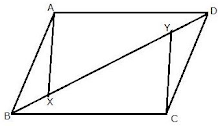Points X and Y are taken on the diagonal BD
such that ∠XAD = ∠YCB = 90°
We need to prove that XA = YC
Proof :
∠XAD = ∠YCB = 90°  [Given]
AD = BC  [Opposite sides of a parallelogram]
∠ADX = ∠CBY  [ Alternate angles]
∴By Angle - Side- Angle criterion of congruence,
The corresponding parts of the congruent triangles are congruent.
∴ XA = YC  [c.p.c.t]
Hence, proved.

7. ABCD is a parallelogram. The sides AB and AD are produced to E and F respectively, such produced to E and F respectively, such that AB = BE and AD = DF.

Prove that: Δ BEC=Δ DCF.

ABCD is a parallelogram. The sides AB and AD are produced to E and F respectively, Such that AB = BE and AD = DF.
We need to prove that △BEC ≅ △DCF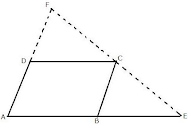Proof :
AB = DC [Opposite sides of a parallelogram ] ...(1)
AB = BE  [Given] ...(2)
From (1) and  (2), we have
BE = DC ...(3)
AD = BC [Opposite sides of a parallelogram] ...(4)
From  (4) and (5), we have
BC = DF  ....(6)
Since AD ∥ BC, the corresponding angles are equal.
∴ ∠DAB = ∠CBE  ...(7)
Since AB ∥ DC, the corresponding angles are equal.
∴ ∠DAB = ∠FDC ....(8)
From (7) and (8), we have
∠CBE = ∠FDC ...(9)
In △BEC and △DCF
BE = DC  [from (3)]
∠CBE = ∠FDC  [from (9)]
BC = DF  [from (6)]
∴ By Side - Angle - Side criterion of congruence,
△BEC ≅ △DCF
Hence, proved.

8. In the following figures, the sides AB and BC and the median AD of triangle ABC are equal to the sides PQ and QR and median PS of the triangle PQR. Prove that ABC and PQR are congruent.

Since, BC = QR, we have
BD = QS  and DC = SR  [D is the midpoint of BC and S is the midpoint of QR]
In △ABD and △PQS
AB = PQ ...(1)
BD = QS ...(3)
Thus, by Side - Side - Side criterion of congruence,
We have △ABD  ≅ △PQS
AC = PR ...(5)
DC = SR ...(6)
Thus, by Side - Side - Side criterion of congruence,
We have
BC = BD + DC [D is the midpoint of BC]
= QS  + SR  [from (3) and (6)]
= QR  [S is the midpoint of QR ] ...(7)
Now consider the triangles △ABC  and △PQR
AB = PQ  [from (1)]
BC = QR  [from (7)]
AC = PR  [from  (7)]
∴ By Side - Side - Side criterion of congruence, we have
△ABC  ≅ △PQR
Hence, proved.

9. In the following diagram, AP and BQ are equal and parallel to each other.

Prove that:

(i) △AOP ≅ △BOQ

(ii) AB and PQ bisect each other.

In the figure, AP and BQ are equal and parallel to each other ,
∴ AP = BQ and AP ∥ BQ .
We need to prove that
(i) △AOP ≅ △BOQ
(ii) AB and PQ bisect each other

Proof:

(i) ∵ AP ∥ BQ
∴ ∠APO  = ∠BQO  [Alternate angles]...(1)
and ∠PAO = ∠QBO  [Alternate angles]...(2)
Now in △AOP and △BOQ,
∠APO = ∠BQO  [from (1)]
AP = BQ  [Given]
∠PAO = ∠QBO  [from (2)]
∴ By Angle - Side - Angle criterion of congruence, we have
△AOP ≅ △BOQ

(ii) The corresponding parts of the congruent triangles are congruent.
∴ OP = OQ  [c.p.c.t]
OA = OB  [c.p.c.t]
Hence AB and PQ bisect each other.

10. In the following figure, OA = OC and AB = BC.
Given :
In the figure, OA = OC, AB = BC
We need to prove that,
(i) ∠AOB = 90°
(ii) △AOD ≅ △COD
Proof:
(i) In  △ABO and △CBO,
AB = BC  [Given]
AO = CO  [Given]
OB = OB  [Common]
∴ By Side - Side - Side criterion of congruence, we have
△ABO ≅ △CBO
The corresponding parts of the congruent triangles are congruent.
∴ ∠ABO = ∠CBO  [c.p.c.t]
⇒ ∠ABD = ∠CBD
and, ∠AOB = ∠COB  [c.p.c.t]
We have
∠AOB + ∠COB = 180° [Linear pair]
⇒ ∠AOB = ∠COB = 90°  and AC ⟂BD
(ii) In △AOD and △COD,
OD = OD  [Common]
∠AOD = ∠COD  [each = 90°]
AO = CO  [given]
∴ By Side - Angle - Side criterion of congruence, we have
△AOD ≅ △COD
(iii) The corresponding parts of the congruent triangles are congruent.
Hence, proved.

11. The following figure shown a triangle ABC in which AB = AC. M is a point on AB and N is a point on AC such that BM = CN.

Prove that:

(i) AM = AN

(ii)triangle AMC = triangle ANB

(iii) BN = CM

(iv) triangle BMC = triangle CNB

In △ABC, AB = AC. M and N are points on AB and AC such that BM = CN.
BN and CM are joined.(i) In △AMC and △ANB
AB = AC  [Given]...(1)
BM = CN  [Given] ...(2)
Subtracting (2) from (1), we have
AB - BM = AC - CN
⇒ AM = AN  ...(3)

(iii) Consider the triangles △AMC and △ANB
AC = AB  [Given]
∠A = ∠A  [common]
AM = AN  [from (3)]
∴ By Side - Angle - Side criterion of congruence, we have
△AMC ≅ △ANB

(ii) The corresponding parts of the congruent triangles are congruent.
∴ CM = BN [c.p.c.t] ...(4)

(iv) Consider the triangles △BMC and △CNB
BM = CN  [given]
BC = BC  [common]
CM = BN  [from (4)]
∴ By Side - Side - Side criterion of congruence, we have
△BMC ≅  △CNB

12. In a triangle ABC, AB = BC, AD is perpendicular to side BC and CE is perpendicular to side AB. Prove that : AD = CE.
AB = BC  (given)
∠B = ∠B  (common angle)
∴ △ABD ≅ △CBE  (by SAS congruence)

13. PQRS is a parallelogram. L and M are points on PQ and SR respectively such that PL= MR. Show that LM and QS bisect each other.

To prove: SP = PQ and MP = PL
Proof:
Since SR and PQ are opposite sides of a parallelogram,
PQ = SR  ...(1)
Also, PL = RM  ...(2)
Subtracting (2) from (1),
PQ - PL = SR - RM
⇒ LQ  = SM  ....(3)
Now, in △ASM and △QLP,
∠MSP = ∠PQL  (alternate interior angles)
∠SMP = ∠PLQ  (alternate interior angles)
SM = LQ  [From (3)]
∴ △SMP ≅ △QLP   (by ASA congruence)
⇒ SP = PQ and MP = PL   (c.p.c.t)
⇒ LM and QS bisect each other.

14. In the following figure, ABC is an equilateral triangle in which QP is parallel to AC. Side AC is produced upto point R so that CR = BP.

Prove that QR bisects PC.

Hint: (Show that ∆QBP is equilateral ⇒ BP = PQ, but BP = CR ⇒ PQ = CR ⇒ ∆ QPM ≅ ∆ RCM)

△ABC is an equilateral triangle.
So, each of its angles equals 60° .
QP is parallel to AC,
⇒ ∠PQB  = ∠RAQ = 60°
In △QBP,
∠PBQ = ∠BQP = 60°
So, ∠PBQ + ∠BQP +∠BPQ = 180° (angle sum property)
⇒ 60° + 60° +∠BPQ = 180°
⇒ ∠BPQ = 60°
So, △BPQ is an equilateral triangle.
⇒QP = BP
⇒QP = CR ...(i)
Now, ∠QPM + ∠BPQ = 180° (linear pair)
⇒∠QPM +60° = 180°
⇒∠QPM = 120°
Also, ∠RCM + ∠ACB = 180° (linear pair)
⇒∠RCM +60° = 180°
⇒∠RCM = 120°
In △RCM and △QMP,
∠RCM = ∠QPM  (each is 120°)
∠RMC = ∠QMP  (vertically opposite angles)
QP = CR  [(from (i)]
⇒ △RCM ≅ △QMP   (AAS congruence criterion)
So, CM = PM
⇒ QR bisects PC.

15. In the following figure, ∠A = ∠C and AB = BC. Prove that ΔABD ≅ ΔCBE.In triangles AOE and COD,
∠A = ∠C  (Given)
∠AOE = ∠COD  (vertically opposite angles)
∴ ∠A + ∠AOE = ∠C + ∠COD
⇒ 180° - ∠AEO = 180° - ∠CDO
⇒ ∠AEO = ∠CDO  ...(i)
Now,
∠AEO + ∠OEB = 180° (linear pair)
And,  ∠CDO +  ∠ODB  = 180° (linear pair)
∴ ∠AEO + ∠OEB =∠CDO +  ∠ODB
⇒ ∠OEB  = ∠ODB [using (i)]
Now, in △ABD and △CBE,
∠A =∠C (given)
AB = BC  (given)
⇒ △ABD ≅ △CBE  (by AAS congruence criterion)

16. AD and BC are equal perpendiculars to a line segment AB. If AD and BC are on different sides of AB prove that CD bisects AB.In △AOD and △BOC,
∠AOD = ∠BOC  (vertically opposite angles)
∠DAO = ∠CBO  (each 90°)
∴ △AOD ≅ △BOC  (by AAS congruence criterion)
⇒ AO = BO  [c.p.c.t]
⇒ O is the mid- point of AB.
Hence, CD bisects AB.

17. In ΔABC, AB = AC and the bisectors of angles B and C intersect at point O.
Prove that:

(i) BO = CO

(ii) AO bisects angle BAC.

[∵ OB and OC are bisectors of ∠B and ∠C respectively, ∠OBC = (1/2)∠B and ∠OCB = (1/2)∠C ] ...(i)
⇒ OB = OC  (Sides opposite to equal angles are equal)...(ii)
Now, in △ABO and △ACO,
AB = AC  (given)
∠OBC  = ∠OCB  [From (i)]
OB = OC  [From (ii)]  (proved)
∴ △ABO ≅ △ACO  (by SAS congruence criterion)
⇒ ∠BAO = ∠CAO  (c.p.c.t)
⇒ AO bisects ∠BAC  (proved)

18. In the following figure, AB = EF, BC = DE and ∠B = ∠E = 90°.Given that, BC = DE
⇒ BC + CD = DE + CD  (Adding CD on both sides)
⇒ BD = CE  ...(i)
Now, in △ABD and △FEC,
AB = EF  (given)
∠ABD = ∠FEC  (Each 90°)
BD = CE    [From (i)]
⇒ △ABD ≅ △FEC   (by SAS congruence criterion)

19. A point O is taken inside a rhombus ABCD such that its distance from the vertices B and D are equal. Show that AOC is a straight line.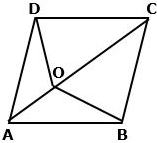In △AOD and △AOB,
AO = AO (common)
OD = OB (given)
⇒ △AOD ≅ △AOB  (by SSS congruence criterion)
⇒ ∠AOD = ∠AOB  (c.p.c.t)...(i)
Similarly, △DOC ≅ △BOC
⇒ ∠DOC = ∠BOC  (c.p.c.t)...(ii)
But, ∠AOB + ∠AOD +∠COD + ∠BOC = 4 Right angles (Sum of the angles at a point is 4 Right angles)
⇒2∠AOD + 2∠COD  = 4 Right angles [Using (i) and (ii)]
⇒ ∠AOD + ∠COD = 2 Right angles
⇒∠AOD + ∠COD = 180°
⇒ ∠AOD and ∠COD form a linear pair.
⇒AO and OC are in the same straight line.
⇒AOC is a straight line.

20. In quadrilateral ABCD, AD = BC and BD = CA. Prove that: